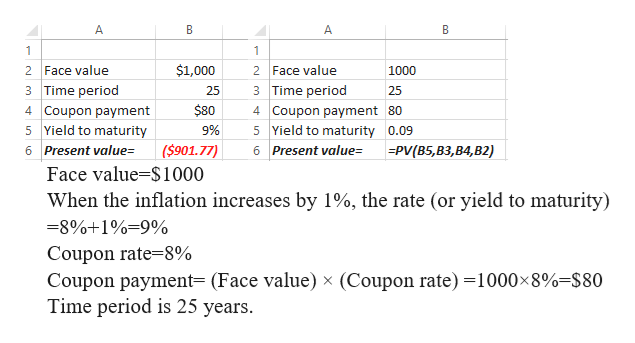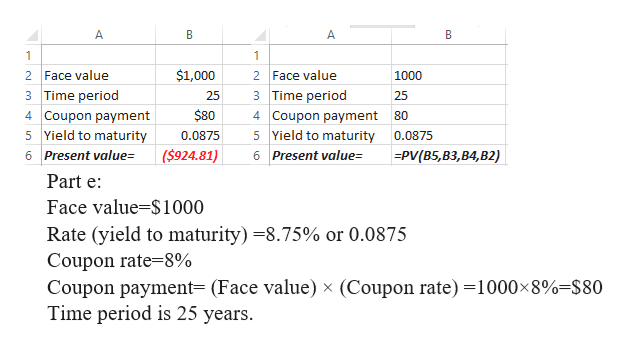# Evaluating Annie​ Hegg's Proposed Investment in Atilier Industries Bonds   Annie Hegg has been considering investing in the bonds of Atilier Industries. The bonds were issued 5 years ago at their \$ 1,000 par value and have exactly 25 years remaining until they mature. They have an 8.0 % coupon interest​ rate, are convertible into 50 shares of common​ stock, and can be called any time at \$ 1,080.00. The bond is rated Aa by​ Moody's. Atilier​ Industries, a manufacturer of sporting​ goods, recently acquired a small​ athletic-wear company that was in financial distress. As a result of the​ acquisition, Moody's and other rating agencies are considering a rating change for Atilier bonds. Recent economic data suggest that expected​ inflation, currently at 5.0 % annually, is likely to increase to a 6.0 % annual rate.Annie remains interested in the Atilier bond but is concerned about​ inflation, a potential rating​ change, and maturity risk. To get a feel for the potential impact of these factors on the bond​ value, she decided to apply the valuation techniques she learned in her finance course.To Doa. If the price of the common stock into which the bond is convertible rises to \$ 30.00 per share after 5 years and the issuer calls the bonds at \$ 1,080.00, should Annie let the bond be called away from her or should she convert it into common​ stock?b. For each of the following required​ returns, calculate the​ bond's value, assuming annual interest. Indicate whether the bond will sell at a​ discount, at a​ premium, or at par value.​(1) Required return is 6.0 %.​(2) Required return is 8.0 %.​(3) Required return is 10.0 %.c. Repeat the calculations in part (b​), assuming that interest is paid semiannually and that the semiannual required returns are​ one-half of those shown. Compare and discuss differences between the bond values for each required return calculated here and in part (b​) under the annual versus semiannual payment assumptions.d. If Annie strongly believes that expected inflation will rise by 1.0 % during the next few​ months, what is the most she should pay for the​ bond, assuming annual​ interest?e. If the Atilier bonds are downrated by​ Moody's from Aa to​ A, and if such a rating change will result in an increase in the required return from 8.0 % to 8.75 %​, what impact will this have on the bond​ value, assuming annual interest?f. If Annie buys the bond today at its \$ 1,000 par value and holds it for exactly 3 years, at which time the required return is 7.0 %​, how much of a gain or loss will she experience in the value of the bond (ignoring interest already received and assuming annual​ interest)?g. Rework part (f​), assuming that Annie holds the bond for 10 years and sells it when the required return is 7.0 %. Compare your finding to that in part (f​), and comment on the​ bond's maturity risk.h. Assume that Annie buys the bond at its current price of \$ 983.80 and holds it until maturity. What will her current yield and yield to maturity (YTM) be, assuming annual​ interest?i. After evaluating all of the issues raised​ above, what recommendation would you give Annie with regard to her proposed investment in the Atilier Industries​ bonds? Please show sub-parts d, e and f.

Question
319 views
Evaluating Annie​ Hegg's Proposed Investment in Atilier Industries Bonds

Annie Hegg has been considering investing in the bonds of Atilier Industries. The bonds were issued 5 years ago at their \$ 1,000 par value and have exactly 25 years remaining until they mature. They have an 8.0 % coupon interest​ rate, are convertible into 50 shares of common​ stock, and can be called any time at \$ 1,080.00. The bond is rated Aa by​ Moody's. Atilier​ Industries, a manufacturer of sporting​ goods, recently acquired a small​ athletic-wear company that was in financial distress. As a result of the​ acquisition, Moody's and other rating agencies are considering a rating change for Atilier bonds. Recent economic data suggest that expected​ inflation, currently at 5.0 % annually, is likely to increase to a 6.0 % annual rate.
Annie remains interested in the Atilier bond but is concerned about​ inflation, a potential rating​ change, and maturity risk. To get a feel for the potential impact of these factors on the bond​ value, she decided to apply the valuation techniques she learned in her finance course.
To Do
a. If the price of the common stock into which the bond is convertible rises to \$ 30.00 per share after 5 years and the issuer calls the bonds at \$ 1,080.00, should Annie let the bond be called away from her or should she convert it into common​ stock?
b. For each of the following required​ returns, calculate the​ bond's value, assuming annual interest. Indicate whether the bond will sell at a​ discount, at a​ premium, or at par value.
​(1) Required return is 6.0 %.
​(2) Required return is 8.0 %.
​(3) Required return is 10.0 %.
c. Repeat the calculations in part (b​), assuming that interest is paid semiannually and that the semiannual required returns are​ one-half of those shown. Compare and discuss differences between the bond values for each required return calculated here and in part (b​) under the annual versus semiannual payment assumptions.
d. If Annie strongly believes that expected inflation will rise by 1.0 % during the next few​ months, what is the most she should pay for the​ bond, assuming annual​ interest?
e. If the Atilier bonds are downrated by​ Moody's from Aa to​ A, and if such a rating change will result in an increase in the required return from 8.0 % to 8.75 %​, what impact will this have on the bond​ value, assuming annual interest?
f. If Annie buys the bond today at its \$ 1,000 par value and holds it for exactly 3 years, at which time the required return is 7.0 %​, how much of a gain or loss will she experience in the value of the bond (ignoring interest already received and assuming annual​ interest)?
g. Rework part (f​), assuming that Annie holds the bond for 10 years and sells it when the required return is 7.0 %. Compare your finding to that in part (f​), and comment on the​ bond's maturity risk.
h. Assume that Annie buys the bond at its current price of \$ 983.80 and holds it until maturity. What will her current yield and yield to maturity (YTM) be, assuming annual​ interest?
i. After evaluating all of the issues raised​ above, what recommendation would you give Annie with regard to her proposed investment in the Atilier Industries​ bonds?

Please show sub-parts d, e and f.
check_circle

Step 1

Part d:
With increase in inflation rate, the rate will also increase by 1%. The inflation adjusted return gives the correct measure of returns.

Step 2

Answer: The most that Annie should pay is \$901.77help_outlineImage TranscriptioncloseA B A B 1 1 \$1,000 2 Face value 2 Face value 1000 3 Time period 4 Coupon payment 5 Yield to maturity 3 Time period 25 25 \$80 4 Coupon payment 80 5 Yield to maturity 0.09 9% (\$901.77) 6 Present value= 6 Present value= PV(B5,B3,B4,B2) Face value-\$1000 When the inflation increases by 1%, the rate (or yield to maturity) -8%+1%=9% Coupon rate-8% Coupon payment= (Face value) x (Coupon rate) 1000x8%=\$80 Time period is 25 years. fullscreen
Step 3

The bond value will decrease to \$924.81 at 8.75% compared to \$1000 at 8% (when ...help_outlineImage TranscriptioncloseA B A B 1 1 2 Face value \$1,000 2 Face value 1000 3 Time period 3 Time period 25 25 \$80 4 Coupon payment 4 Coupon payment 80 5 Yield to maturity 5 Yield to maturity 0.0875 0.0875 (\$924.81) 6 Present value= 6 Present value= =PV(B5,B3,B4,B2) Part e Face value S1000 Rate (yield to maturity) -8.75% or 0.0875 Coupon rate 8% Coupon payment= (Face value) x (Coupon rate) =1000x8%=\$80 Time period is 25 years. co fullscreen

### Want to see the full answer?

See Solution

#### Want to see this answer and more?

Solutions are written by subject experts who are available 24/7. Questions are typically answered within 1 hour.*

See Solution
*Response times may vary by subject and question.
Tagged in